# How to Get Running Totals in Google Sheets (Easy Formula)

Running Totals in Google Sheets: A running total is a valuable aggregation technique that allows you to examine the overall sum of a series of data at any moment in time. It is frequently used in a variety of academic and business applications. In this article, let’s understand how to use the running total function along with important Google Sheet tips. Read on to find out more.

## What is Running Total?

A running total often called a cumulative sum, is a series of partial sums of an integer sequence.

When a new number is introduced to the sequence, it is updated by adding the new number’s value to the previous running total. This allows you to get a sense of the sequence’s progression while also knowing the ultimate sum at a glance by merely looking at the last total.

A running total is commonly used in the business world to keep track of spending, revenue, inventory, and staff hours.

### How to get a Running Total in Google Sheets?

We should use the SUM function to find the running totals in Google Sheets. The steps to create running totals in Google Spreadsheet are listed below:

• Step 1: Select the cell where you would like to apply the running total function.
• Step 2: Now type the formula as =SUM(\$B\$2:B2).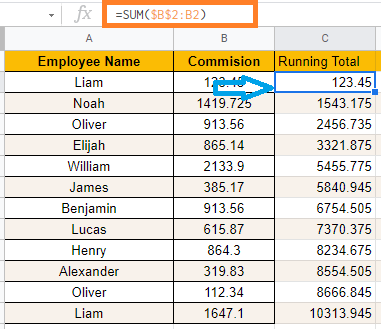• Step 3: Now drag the fill handle to apply the formula to all the cells.
• Step 4: You will see the results.

### How Running Totals works in Google Sheets?

The formula we have used here is SUM(\$B\$2:B2). This adds the values from Cell B2 to B2.Now when you drag the formula applied cell to the next cell, this adds the values from Cell B2 to B3.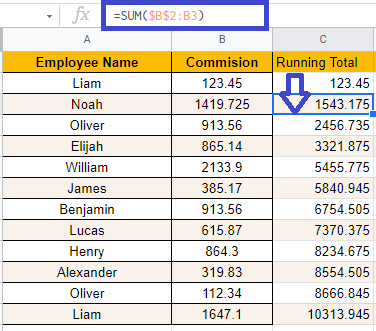Next, when the formula is applied to the next cell, the function adds the values from B2 to B4.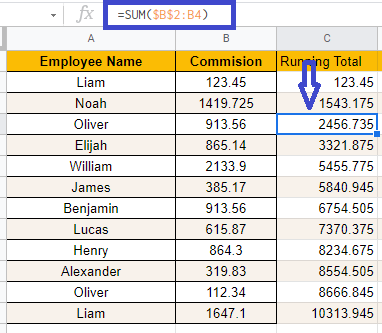The sum of all values from the first to the last row of the list is calculated in the last row.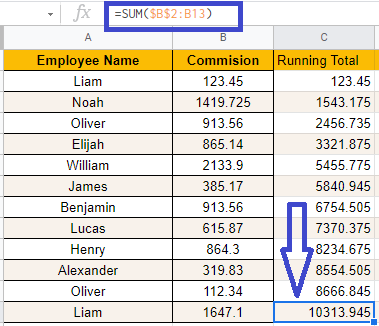### How to Create Dynamic Running Column?

If you don’t want to copy the Running total formula every time a new value is added to the list, you may add an IF statement to the formula to ensure that a running total is calculated only if the matching daily expenditure value isn’t blank.

For example, the formula for cell C2 may be altered as

=IF(B2<>””,SUM(\$B\$2:B2),””)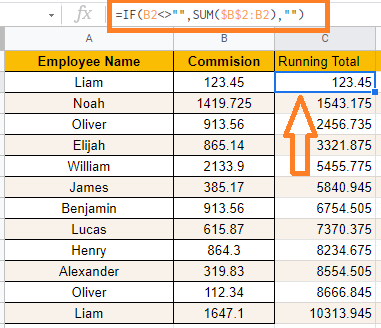You might then copy this formula to the complete column ahead of time so that only when a new number is entered in column B, the matching value in column C calculates the running total up to that point.